# Detrending auto-correlated data¶

Here, we demonstrate spurious cross-correlations originating from auto-correlated time-series. We then show how taking correlations between detrended versions of these time series does not lead to falsely significant correlations. Our example revolves around two random walks, which one might intuitively believe to be independent. However, any two random walks (if long enough) are almost always correlated with extremely low p-values. This is due in part to random walks' autocorrelation, meaning that their position at any time is highly dependent on their position at the previous time step. Below, we show 1) how two random walks exhibit strong cross-correlations, as well as individual auto-correlations. We then show 2) how removing trends leads to non-significant correlations.

## 1. Generate two random walks.¶

Intuititively, one would not think that two random walks should show a temporal correlation. But, due to auto-correlative effects, they often do.

In :
import pylab as pl
import scipy.stats as ss

In :
# simulate two random walks using a series of random coinflips. the random walk is the cumulative sum of the number of heads (+1)
# or tails (-1) flipped.
np.random.seed(1)
coinflip1_v = sign(1*(np.random.random(1000) > 0.5)-0.5)
coinflip2_v = sign(1*(np.random.random(1000) > 0.5)-0.5)
x1_v = cumsum(coinflip1_v)
x2_v = cumsum(coinflip2_v)


Visualize the series of coinflips, and the resultant random walks

In :
pl.figure(figsize=(24,2))
pl.plot(coinflip1_v)
pl.ylim([-1.5,1.5])
pl.xlabel('Coinflip')
pl.title('Coinflips #1');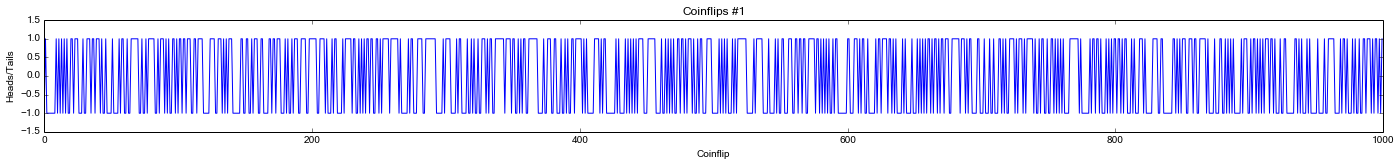In :
pl.figure(figsize=(24,2))
pl.plot(coinflip2_v,'r')
pl.ylim([-1.5,1.5])
pl.xlabel('Coinflip')
pl.title('Coinflips #2');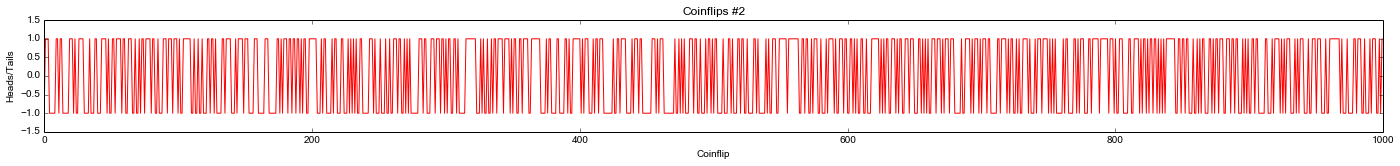Visualize the random walks based on the coinflips. Position is based on the preceding total number of heads (+1) and tails (-1) coinflips. Do these look correlated?

In :
pl.figure()
pl.plot(x1_v,label='Walk #1')
pl.plot(x2_v,'r',label='Walk #2')
pl.grid()
pl.title('Random walks')
pl.legend(loc=2)
pl.xlabel('Coinflip')
pl.ylabel('Position');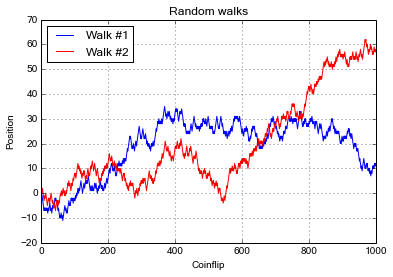The cross-correlation though, may be surprising

In :
xcorr_t = ss.pearsonr(x1_v,x2_v)
print "Random walk cross-correlation (rho): %1.2f" % xcorr_t
print "Random walk cross-correlation (pval): %1.4e" % xcorr_t

Random walk cross-correlation (rho): 0.31
Random walk cross-correlation (pval): 8.8596e-24


This surprising cross-correlation is associated with the auto-correlative nature of the random walks. Below, we illustrate and measure this auto-correlation.

In :
pl.figure()
pl.plot(x1_v[:-1],x1_v[1:],'o')
pl.title('Walk #1')
pl.xlabel('Position at Coinflip i')
pl.ylabel('Position at Coinflip i+1')

pl.figure()
pl.plot(x2_v[:-1],x2_v[1:],'or')
pl.title('Walk #2')
pl.xlabel('Position at Coinflip i')
pl.ylabel('Position at Coinflip i+1');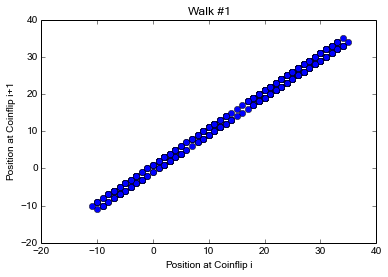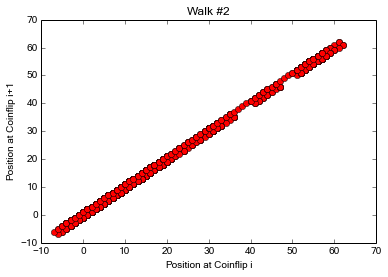In :
print "Random walk 1 auto-correlaton (rho): %1.2f" % ss.pearsonr(x1_v[:-1],x1_v[1:])
print "Random walk 2 auto-correlaton (rho): %1.2f" % ss.pearsonr(x2_v[:-1],x2_v[1:])

Random walk 1 auto-correlaton (rho): 1.00
Random walk 2 auto-correlaton (rho): 1.00


## 2. Detrending the random walks.¶

A common strategy for resolving the apparent cross-correlation between auto-correlated time-series, is to remove the trends within each time-series and instead study residual time-series. Below, we use ARIMA models (a common time-series tool) to detrend our random walks, since this is the tool we use in the manuscript. However, we note that if you knew all your time-series were random walks, a simpler method would be to just take the difference between neighboring time-points.

In :
# this example calls R code. to run it, you'll need to install R, the rpy2 Python module, and the 'forecast' R package.
import rpy2.robjects as R
import rpy2.robjects.numpy2ri
rpy2.robjects.numpy2ri.activate()
R.r('library(forecast)');

Loading required package: zoo

Attaching package: ‘zoo’

The following objects are masked from ‘package:base’:

as.Date, as.Date.numeric

This is forecast 5.3


In :
R.r.assign('x1_v',x1_v)
R.r.assign('x2_v',x2_v)

R.r('x1_arima<-auto.arima(x=x1_v,max.p=2,d=1,max.q=2,ic="bic",approximation=FALSE)');
R.r('x2_arima<-auto.arima(x=x2_v,max.p=2,d=1,max.q=2,ic="bic",approximation=FALSE)');

residual1_v = np.array(R.r('x1_arima$residuals')) residual2_v = np.array(R.r('x2_arima$residuals'))


The residual time-series resemble the series of coinflips that gave rise to the random walk.

In :
pl.figure(figsize=(24,2))
pl.plot(residual1_v)
pl.ylim([-1.5,1.5])
pl.xlabel('Coinflip')
pl.ylabel('Residual')
pl.title('Residuals #1');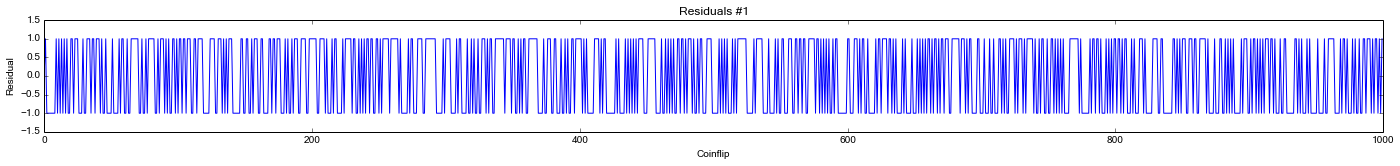In :
pl.figure(figsize=(24,2))
pl.plot(residual2_v,'r')
pl.ylim([-1.5,1.5])
pl.xlabel('Coinflip')
pl.ylabel('Residual')
pl.title('Residuals #2');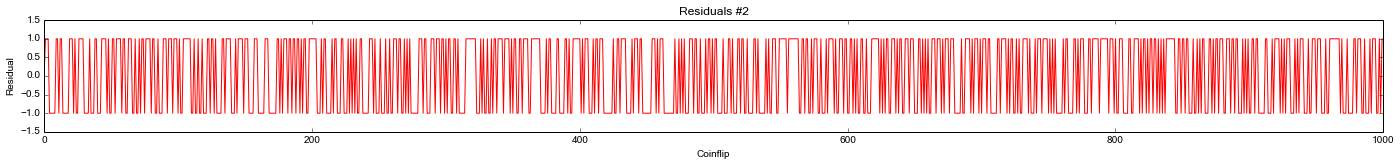The residual time-series should not be autocorrelated

In :
print "Residual 1 auto-correlaton (rho): %1.3f" % ss.pearsonr(residual1_v[:-1],residual1_v[1:])
print "Resdiual 2 auto-correlaton (rho): %1.3f" % ss.pearsonr(residual2_v[:-1],residual2_v[1:])

Residual 1 auto-correlaton (rho): -0.058
Resdiual 2 auto-correlaton (rho): -0.005


And, the cross-correlation of the random walks' residuals should not be correlated either. In our manuscript, we generally perform these kinds of cross-correlations (ones between residual time-series).

In :
xcorr_t = ss.pearsonr(residual1_v,residual2_v)
print "Random walk cross-correlation (rho): %1.2f" % xcorr_t
print "Random walk cross-correlation (pval): %1.2f" % xcorr_t

Random walk cross-correlation (rho): 0.02
Random walk cross-correlation (pval): 0.61